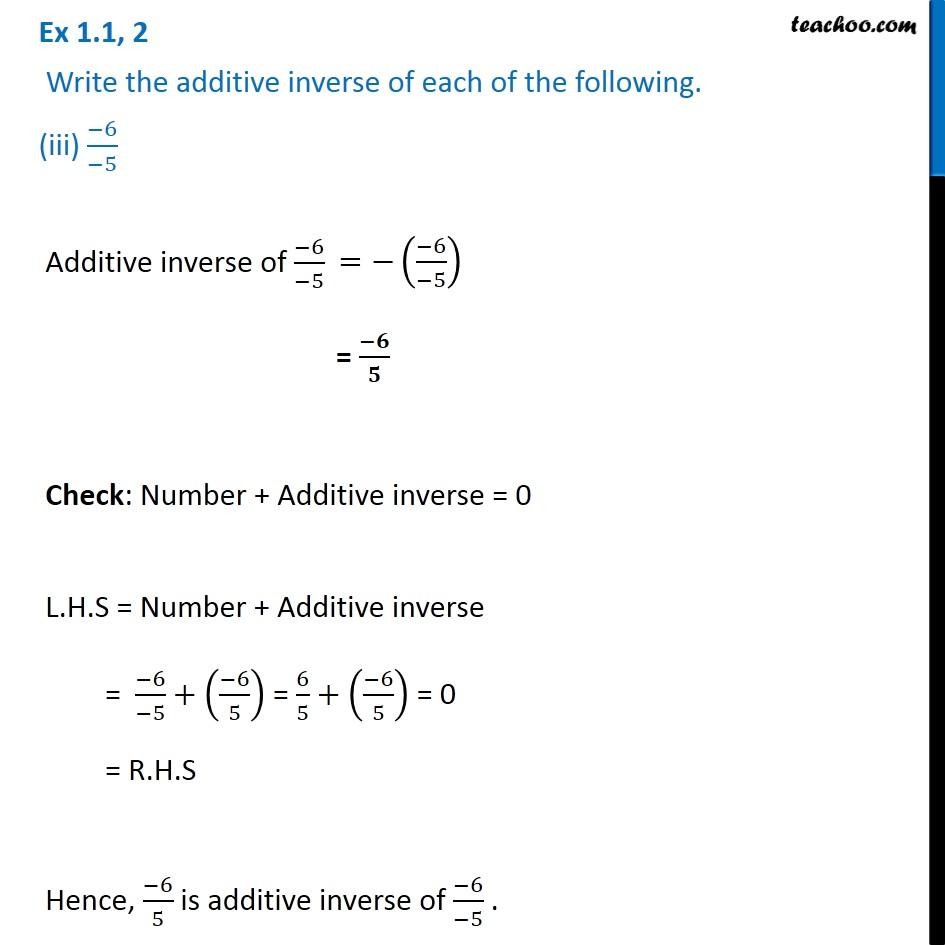Ex 1.1

Chapter 1 Class 8 Rational Numbers
Serial order wiseLearn in your speed, with individual attention - Teachoo Maths 1-on-1 Class

### Transcript

Ex 1.1, 2 Write the additive inverse of each of the following. (iii) ( 6)/( 5) Additive inverse of ( 6)/( 5) = ( )/ Check: Number + Additive inverse = 0 L.H.S = Number + Additive inverse = ( 6)/( 5)+(( 6)/5) = 6/5+(( 6)/5) = 0 = R.H.S Hence, ( 6)/5 is additive inverse of ( 6)/( 5) .